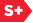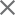### Proceedings Paper

Improved time bounds for near-optimal sparse Fourier representations
Author(s): A. C. Gilbert; S. Muthukrishnan; M. Strauss
Format Member Price Non-Member Price
PDF \$17.00 \$21.00

Paper Abstract

•We study the problem of finding a Fourier representation R of m terms for a given discrete signal A of length N. The Fast Fourier Transform (FFT) can find the optimal N-term representation in time O(N log N) time, but our goal is to get sublinear time algorithms when m << N. Suppose ||A||2M||A-Ropt||2, where Ropt is the optimal output. The previously best known algorithms output R such that ||A-R||22≤(1+ε))||A-Ropt||22 with probability at least 1-δ in time* poly(m,log(1/δ),log N,log M,1/ε). Although this is sublinear in the input size, the dominating expression is the polynomial factor in m which, for published algorithms, is greater than or equal to the bottleneck at m2 that we identify below. Our experience with these algorithms shows that this is serious limitation in theory and in practice. Our algorithm beats this m2 bottleneck. Our main result is a significantly improved algorithm for this problem and the d-dimensional analog. Our algorithm outputs an R with the same approximation guarantees but it runs in time m•poly(log(1/δ),log N,log M,1/ε). A version of the algorithm holds for all N, though the details differ slightly according to the factorization of N. For the d-dimensional problem of size N1 × N2 × •• × Nd, the linear-in-m algorithm extends efficiently to higher dimensions for certain factorizations of the Ni's; we give a quadratic-in-m algorithm that works for any values of Ni's. This article replaces several earlier, unpublished drafts.

Paper Details

Date Published: 21 September 2005
PDF: 15 pages
Proc. SPIE 5914, Wavelets XI, 59141A (21 September 2005); doi: 10.1117/12.615931
Show Author Affiliations
A. C. Gilbert, Univ. of Michigan (United States)
S. Muthukrishnan, Rutgers Univ. (United States)
M. Strauss, Univ. of Michigan (United States)

Published in SPIE Proceedings Vol. 5914:
Wavelets XI
Manos Papadakis; Andrew F. Laine; Michael A. Unser, Editor(s)